## Saturday, 19 November 2011

### CBSE Class9-Science 11 (Work, Power Energy) MCQ

Work, Power Energy
MCQs

Q1: An example of Kinetic energy would be:
a. a moving car
b. a charge particle in an electric field.
c. a stretched rubber band just released.
d. all of the above.

Q2: Newton-meter is the SI unit of
a. acceleration
b. work
c. power
d. force

Q3: Which of the following is/are example(s) of Potential Energy
a. A moving car.
b. A battery
c. A book resting on the table
d. both b and c

Q4: The other name of Nm is
a. ampere
b. volt
c. joule
d. hertz

Q5: kWh is the unit of
a. acceleration
b. work
c. power
d. energy

Q6: Which of the following is NOT correct?
a. energy is the ability of doing work
b. Work can be expressed as Force x Displacement
c. unit of power is Joule.
d. power is the amount of work done per unit of time

Q7: A body of mass 3 kg is dropped from the height of 1m. The kinetic energy of the body will be when it touches the ground.
a. 29.4 N
b. 29.4 J
c. 150 N
d. 150 J

Q8: Two objects of masses 1Kg and 3Kg have equal momentum. What is the ratio of their kinetic energies?
a. 3:1
b. 9:1
c. 1:1
d. 1:2

Q9: Considering air resistance negligible, the sum of potential and kinetic energies of the free falling body would be
a. zero
b. would increase
c. would decrease
d. remains fixed.

Q10: A 1-kg block is lifted vertically 1m by a boy. The work done by the boy is:
a. 1 J
b. 0 J
c. 9.8 J
d. 0.1 J

Q11: Two bodies of masses m1 and m2 have equal kinetic energies.  If  p1 and p2 are their respective momentum, then ratio p1 : p2 is:
a. m1 : m2
b. m2 : m1
c. √m1 : √m2
d. m12 : m22

Q12: A light and heavy body have equal momenta. Which one has greater kinetic energy?

a. the light body
b. the heavy body
c. both have same K.E.
d. none of these

Answers:  1-d     2-b     3-d     4-c   5-d     6-c    7-b   8-a   9-d   10-c    11-c (Hint)   12-a#### 12 comments:

1.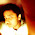Q6. option a. energy is the rate of doing work is also wrong with option c. as power is the rate of doing work.

1.Thanks Fahim for the correction. Option 'a' is redefined as "Thanks Karim for the correction. It is redefined as "energy is the ability of doing work"

2.per unit of time also means rate of doing work

2.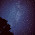Question 11 is wrong. The answer is the square root of m2 to the square root of m1.

1.it is correct

3.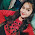imp ques thanks

4.thanks for the help

5.this was in my text too

6.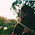not so good questions and wrong answers
dont make us fool

7.which is wrong nd which is true....... its very confusing

8.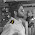gr8 helped a lot

9.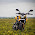How to do question 10 ?

We love to hear your thoughts about this post!